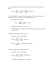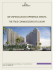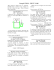# Solution

## Transcription

Solution
```MAE 108 - Probability and Statistical Methods for Engineers - Spring 2015
Midterm 2, May 22
Instructions
(i) One cheat sheet with notes, book tables, and calculator with no communication capabilities are
allowed,
(ii) No cellphones, tablets, or laptops,
(iii) You have 50 minutes,
(v) Do not forget to write your name and student number in your exam.
Questions
1. (7 points) The arrival of customers at a store can be modeled as a Poisson distribution. Suppose that
between 8:00 and 9:00 a.m. an average of 6 customers arrive at the store.
(i) What is the probability that exactly 2 customers arrive between 8:00 and 8:30a.m.?
(ii) Suppose that the service time T of a customer at the store follows an exponential distribution. If
the mean service time is 10 minutes, what is the probability that a customer has to wait less than
5 minutes to be serviced?
Solution:
(i) Let X be the number of customers arriving at the store from 8:00am to 8:30a.m. We are
told X is distributed as a Poisson process with parameter λ = v ∗ t. Given the data of the
problem, we have that v = 6/60 customers per minute and t = 30 minutes. Thus, λ = 3.
(+ 2 points)
The probability that exactly 3 customers arrive at the store is
P (X = 2) =
λ2 −λ 32 −3
e = e = 0.2240
2!
2!
(+ 1 point)
(each computation error is discounted as -0.5)
(ii) If the service time is T is distributed as an exponential, we have that
fT (t) = µe−µt ,
t≥0
where µ is equal to the inverse of the mean service time. In other words, µ = 1/10.
(+ 2 points)
Then, the probability that a customer has to wait less than 5 minutes to be serviced is
Z 5
Z 5
1 −t/10
P (T < 5) =
fT (t)dt =
e
= 1 − e−5/10 = 1 − e−0.5 = 1 − 0.6067 = 0.3932
0
0 10
(+ 2 points)
2. (9 points) Consider the function f (x) = c e−(x−18)/6 , for x ≥ 0. Let X be a random variable distributed
as f (x).
(i) Find the value of c and the mean value of X.
(ii) What is the probability that X < 20?
(iii) If we reproduce 4 independent trials of an experiment in which we observe realizations of X,
what is the probability of finding exactly 3 times that X < 20?
Solution:
(i) The value of c follows from
R +∞
0
ce−(x−18)/6 dx = 1.
(+1 point)
We have that
Z
+∞
0
c e−(x−18)/6 dx = c[−6e−(x−18)/6 ]+∞
= c ∗ 6 ∗ e3 = 1
0
Therefore, c = e−3 /6 = 0.0082.
(+ 1 point)
The mean value of X is given by
Z
+∞
Z
+∞
x∗c∗e
dx = c ∗ e ∗
x ∗ e−x/6 dx =
0
0
Z +∞
−x/6
3
−x/6 +∞
e
dx
= c ∗ e [−6 ∗ x ∗ e
]0 + 6
E[X] =
−(x−18)/6
3
0
=6
(+ 2 points)
(ii) This probability is given by
20
Z
P (X < 20) =
−20/6
ce−(x−18)/6 dx = −6 ∗ c ∗ e3 ∗ [e−x/6 ]20
= 0.9643
0 =1−e
0
(+ 2 points)
(iii) By considering the 4 trials to be a Bernoulli sequence, then we can assume the number of
times we get X < 20, N , is described by a Binomial with parameters p = 0.9643 and n = 4.
(+ 1 point)
Then,
P (N = 3) = 4 ∗ (0.9643)3 (1 − 0.9643) = 0.1280
(+ 2 points)
Page 2
3. (12 points) The finished inside diameter of a piston ring is normally distributed with a mean of 10
centimeters and a standard deviation of 0.03 centimeter.
(i) What proportion of rings will have inside diameters exceeding 10.075 cm?
(ii) What is the probability that a piston ring will have an inside diameter between 9.97 and 10.03
cm?
(iii) Below what value of inside diameter will 15% of the piston rings fall?
Solution:
(i) For a Gaussian distribution of mean µ = 10 and standard deviation σ = 0.03, we have
10.075 − 10
= 1 − Φ(2.5)
P (d > 10.075) = 1 − P (d ≤ 10.075) = 1 − Φ
0.03
(+ 3 points)
Using the table of Appendix A.1,
P (d > 10.075) = 1 − 0.9938 = 0.0062
(+ 1 point)
(ii) Similarly,
P (9.97 ≤ d ≤ 10.03) = Φ
10.03 − 10
9.97 − 10
−Φ
= Φ(1)−Φ(−1) = 2Φ(1)−1 = 0.6826
0.03
0.03
(+ 4 points)
(iii) We wish to find D such that
P (d ≤ D) = Φ
D − 10
0.03
= 0.15
(+ 1 point)
Let’s define x = (D − 10)/0.03. Clearly we expect x < 0, so
1 − Φ(−x) = 0.15,
i.e. Φ(−x) = 1 − 0.15 = 0.85
(+ 1 point)
Using the table, we find −x ≈ 1.04. Therefore,
10 − D
= 1.04,
0.03
i.e.
D = 0.969
(+ 2 points)
Page 3
4. (7 points) The length of time, in seconds, that a computer user takes to read his or her email is distributed as a lognormal random variable with mean µ = 1.8 and variance σ 2 = 4.0. What is the
probability that a user reads email for more than 20 seconds?
Solution:
The parameters of the lognormal distribution are given by:
"
2 #
4.0
σ
2
= ln 1 +
= 0.804
ζ = ln 1 +
µ
1.82
i.e.
ζ = 0.897
(+ 2 points)
and
1
λ = ln µ − ζ 2 = ln 1.8 − 0.402 = 0.186
2
(+ 1 point)
The desired probability is then given by
P (T > 20) = 1 − P (T ≤ 20) = 1 − Φ
ln 20 − λ
ζ
=1−Φ
2.81
0.897
= 1 − Φ(3.13)
(+ 3 points)
Using the table of appendix A.1, we find:
P (T > 20) = 1 − 0.999 = 0.001
(+ 1 point)
Page 4
```

### key - Ramanujan & Raman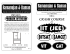### unfinished project - Western North Carolina Commercial Property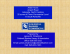### Log Max 928A - Biomass Innovation Centre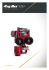### Suggested problems Chapter 14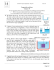### Document 6574678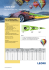### 3.25 Refrigerant 134a in a pistonâcylinder assembly undergoes a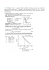### Piston Insertion Tool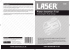### 1. A certain sample of brass has a mass of... density? kg